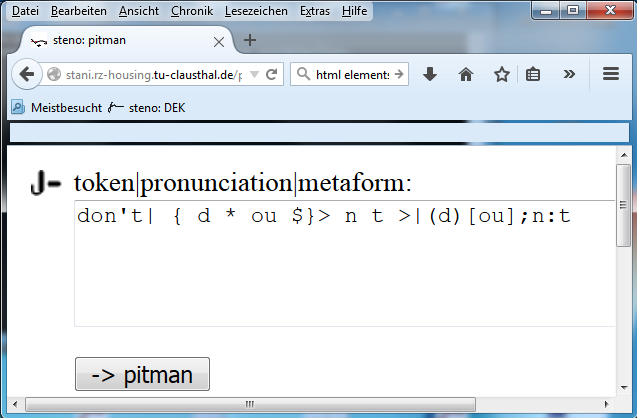## Using Steno Fonts in LaTeX

Suppose You want to obtain LaTeX output like this one pdf-file.

\documentclass[11pt,a5paper]{article}
\setlength{\parindent}{0in}
\begin{document}
The day is short\\
the night is long\\
...
\end{document}

Add the following (red marked) lines:

\documentclass[11pt,a5paper]{article}
\pdfpkresolution=1200
\newcommand{\st}{
{\sF{\symbol{#2}}
}}
\font\sF = sf0 scaled 1000

\setlength{\parindent}{0in}
\begin{document}
\st{The}{} \st{day}{} \st{is}{} \st{short}{}\\
The day is short\\
\st{the}{} \st{night}{} \st{is}{} \st{long}{}\\
the night is long\\
\st{why}{} \st{do}{} \st{we}{} \st{work}{} \st{so}{} \st{hard}{}\\
why do we work so hard\\
\st{to}{} \st{get}{} \st{what}{} \st{we}{} \st{dont}{} \st{even}{} \st{want}{}\st{.}{}\\
to get what we don't even want.\\

\begin{center}
\begin{tabular}{l|l|l}
&high&higher\\ \hline
Pitman 2000&\st{high}{}&\st{higher}{}\\
Pitman New Era&\st{nehigh}{}&\st{nehigher}{}\\

\end{tabular}
\end{center}

\end{document}

Send Your tex-file to abouter.php to obtain files named "13.tex", "13.steni", "13.tfm" and "13.1200pk". (If You are not lucky enough then the "13" will be another number). Observe the lines
\st{The}{} \st{day}{2} \st{is}{10} \st{short}{15}
\st{the}{17} \st{night}{14} \st{is}{10} \st{long}{11}
...

in Your 13.tex-file. The numbers following the words in "\st"-commands (e.g. "17" in "\st{the}{17}") are the character codes in the Pitman 2000 steno font named 13. Observe that the line
\font\sF = sf0 scaled 1000

was changed into
\font\sF = 13 scaled 1000

Now You can try in Your LaTeX-environment the comand "pdflatex 13.tex". You will obtain something like this.

The Pitman stenem for the first "The" is missing --- obviously we have to add the code number:

\st{The}{17} \st{day}{2} \st{is}{10} \st{short}{15}
\st{the}{17} \st{night}{14} \st{is}{10} \st{long}{11}
...


We also don't like the stenem for "don't". try with Proof=7 and the input "don't" yields:Now change in "13.steni" the metaform in the line

dont| { d o n t }|(d)[ou];n:t

The Pitman New Era metaforms for "high" and "higher" in the same file
nehigh| { n e h i g h }|,s(ch,r)[ai]
nehigher| { n e h i g h e r }|,s(ch,r)[ai_]&(_r)


are a little cryptic, please read Writing Pitman shorthand with Metafont and LaTeX and try it interactively.

Now You can send the so corrected "13.steni" to abouter.php and You will obtain corrected font files "?.tfm" and "?.1200pk".